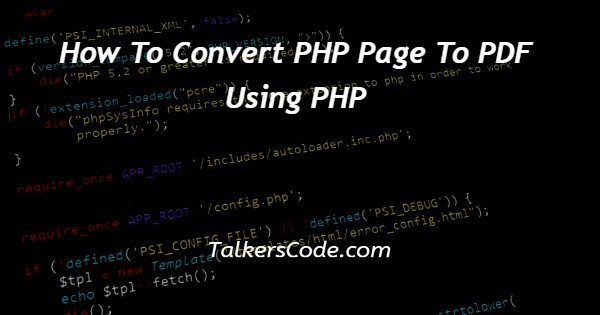# How To Convert PHP Page To PDF Using PHP

Last Updated : Jan 1, 2023In this tutorial we will show you the solution of how to convert PHP page to pdf using PHP, I like these types of concept very much. Let us understand this first.

For example, like in a php webpage we fetch data from our database in which we display content of particular user with sequence and a button of download pdf under each user detail.

Now, after clicking on specific button the data of specific user must be downloaded in pdf form. Now, let us understand the example below.

## Step By Step Guide On How To Convert PHP Page To PDF Using PHP :-

• In first step, we create php page with content and a button.
• In next step, we have to create a php file for make pdf format.

Let, us see the below codes that how to use above steps.

```<?php
\$servername = "localhost";
\$databasename = "itsdatabase";
\$conn = mysqli_connect(\$servername,
?>
<html>
<title>
How to convert php page to pdf using php
</title>
<body>
<?php
if (!\$conn) {
die("Connection failed: " . mysqli_connect_error());
}
\$query = "SELECT * FROM `dataTable`;";
\$result = mysqli_query(\$conn, \$query);
if (mysqli_num_rows(\$result) > 0) {
while(\$row = mysqli_fetch_assoc(\$result)) {
echo "Student : " . \$row["name"]
. " is studying in class " . \$row["class"].. " scores " . \$row["marks"]. "marks <br>";
?>
</a>
<?php
}
} else {
echo "0 results";
}
\$conn->close();
?>
</body>
</html>
// next php file that is pdfFile.php
<?php
\$servername = "localhost";
\$databasename = "itsdatabase";
\$conn = mysqli_connect(\$servername,
\$id = \$_request[‘id’];
\$query = "SELECT * FROM `dataTable` where id = \$id";
\$result = mysqli_query(\$conn, \$query);
namespace Dompdf;
if (mysqli_num_rows(\$result) > 0) {
while(\$row = mysqli_fetch_assoc(\$result)) {
\$name = \$row[“name”];
\$class= \$row[“name”];
\$marks = \$row[“name”];
if(isset(\$_POST['button']))
{
\$dompdf = new Dompdf();
?>
<table border=1 align=center width=400>
<tr><td>Name : </td><td>'.<?php echo \$name ?>.'</td></tr>
<tr><td>Class : </td><td>'.<?php echo \$class ?>.'</td></tr>
<tr><td>Marks : </td><td>'.<?php echo \$marks ?>'</td></tr>
</table>
<?php
');
\$dompdf->setPaper('A4', 'landscape');
\$dompdf->render();
\$dompdf->stream("",array("Attachment" => false));
exit(0);
}
?>```
1. As, we see above that we fetch data from database and echo or display that in a php webpage. Here, we also create a dynamic button in which we store the id of specific user also.
2. At next on click on anchor tag next page opens which gets id from previous page, you can see the anchor tag for reference that how to send data from one page to another. There is also an article on how to send dynamic data from one page to another in php.
3. At next page, we fetch data of particular or say required user form database and uses the dompdf library. The dompdf library must be present at your required folder.
4. Now, we set basic configuration of dompdf library in our page and the data that we fetch from database, use in dompdf code to render a pdf. You can see the above code to see how to use dompdf in php.

## Conclusion :-

At last in conclusion, here we can say that with the help of this article we are able to understand how to convert php page to pdf using php.

I hope this tutorial on how to convert PHP page to pdf using PHP helps you and the steps and method mentioned above are easy to follow and implement.